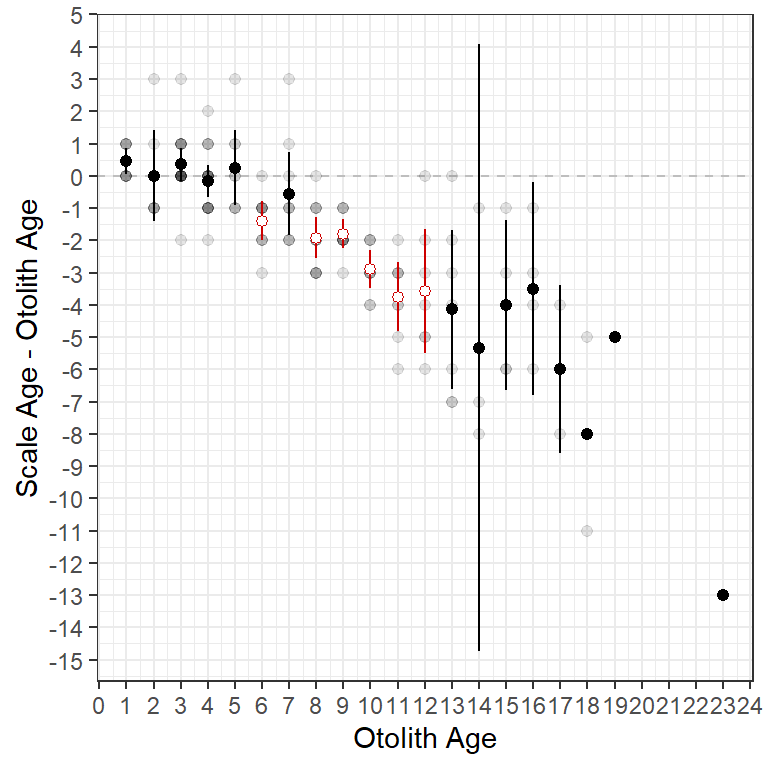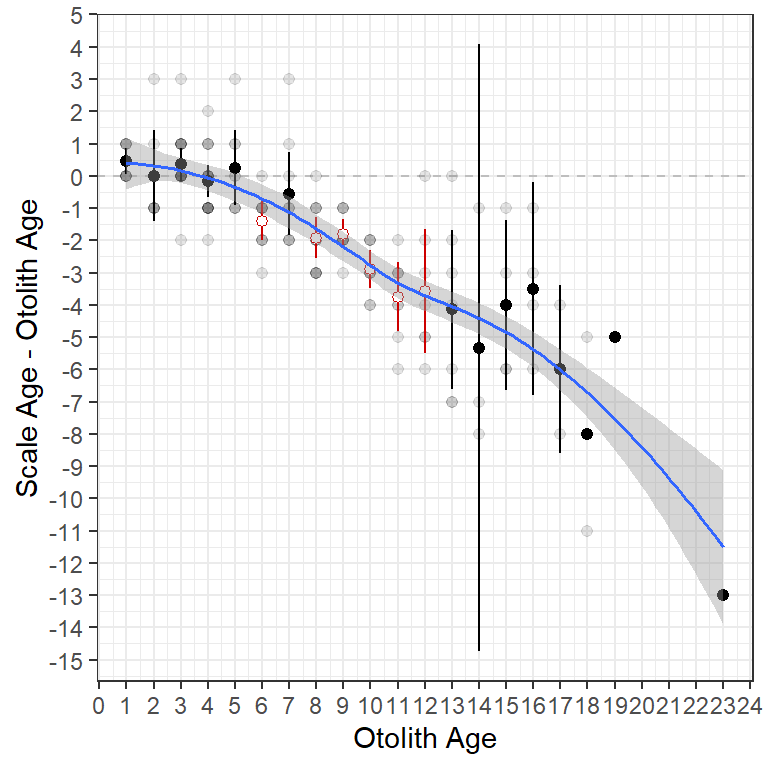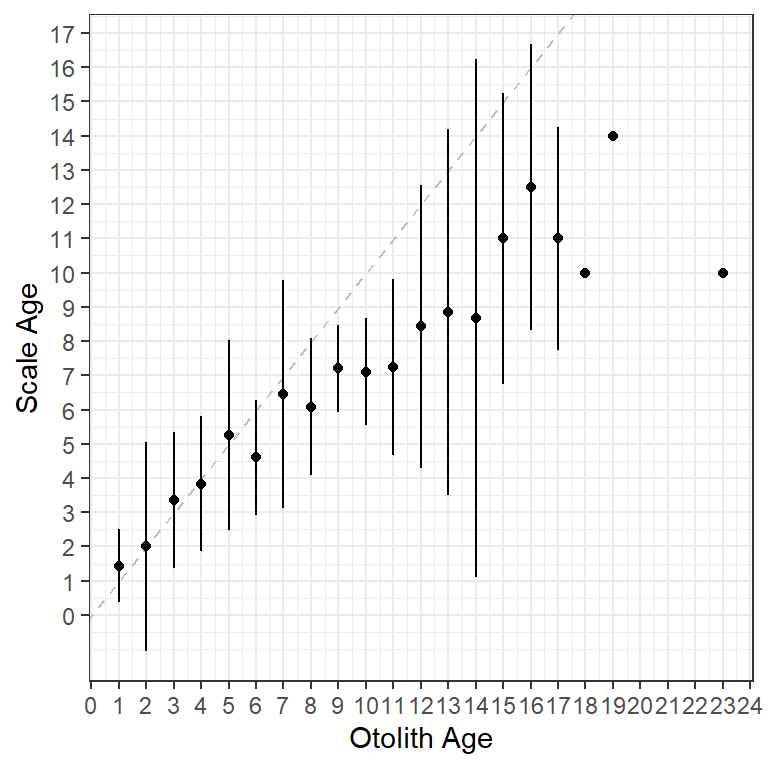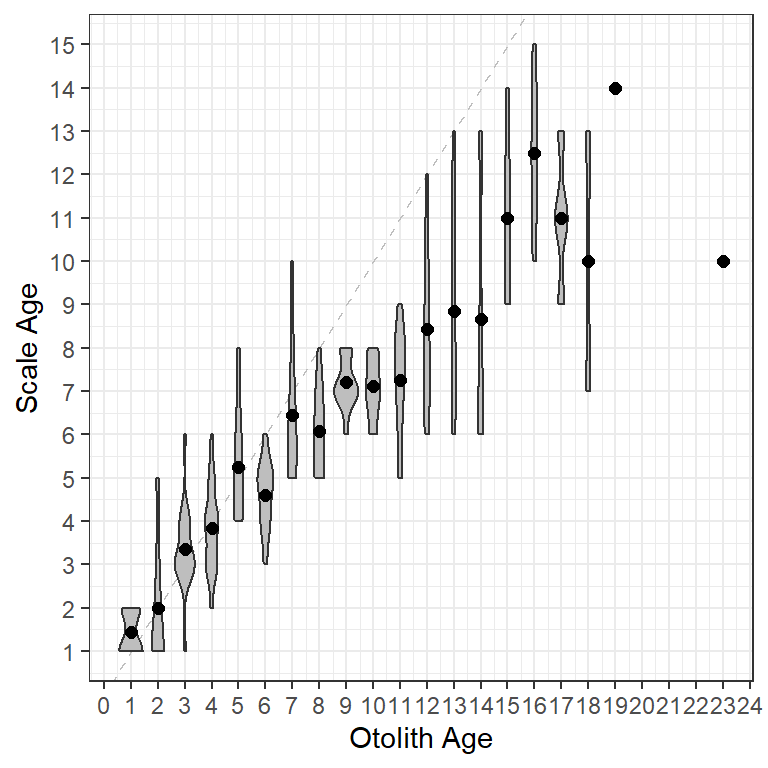# Age Bias Plots in ggplot2

Flexibly construct age bias and age difference plots using ggplot2
Age
Age Comparisons
ggplot2
Author

Michael Lant

Published

Mar 15, 2021

Modified

Dec 7, 2022

Note

The following packages are loaded for use below. I also set the default `ggplot` theme to `theme_bw()` for a classic “black-and-white” plot (rather than the default plot with a gray background).

``````library(FSA)          # for WhitefishLC, ageBias(), plotAB()
library(dplyr)        # for mutate()
library(ggplot2)
theme_set(theme_bw())``````

# Introduction

Age-bias and difference plots can be constructed with `plot()` and `plotAB()` in the `FSA` package. However, these types of plots can be more flexibly constructed using `ggplot2`. Below I will use `ggplot2` to recreate many of the plots shown in the documentation for `plot()` and `plotAB()`.

# Data

The `WhitefishLC` data frame from `FSA`.1 contains age readings made by two readers on scales, fin rays, and otoliths, along with consensus readings for each structure.

• 1 These data are loaded automatically with `library(FSA)`.

• ``head(WhitefishLC)``
``````#R|    fishID  tl scale1 scale2 scaleC finray1 finray2 finrayC otolith1 otolith2
#R|  1      1 345      3      3      3       3       3       3        3        3
#R|  2      2 334      4      3      4       3       3       3        3        3
#R|  3      3 348      7      5      6       3       3       3        3        3
#R|  4      4 300      4      3      4       3       2       3        3        3
#R|  5      5 330      3      3      3       4       3       4        3        3
#R|  6      6 316      4      4      4       2       3       3        6        5
#R|    otolithC
#R|  1        3
#R|  2        3
#R|  3        3
#R|  4        3
#R|  5        3
#R|  6        6``````

Intermediate and summary statistics for the comparison of paired ages (e.g., between consensus scale and otolith ages) can be extracted from the objected returned by `ageBias()` from `FSA`.2

• 2 As described in the documentation.

• ``````ab1 <- ageBias(scaleC~otolithC,data=WhitefishLC,
ref.lab="Otolith Age",nref.lab="Scale Age")``````

For example, the `\$data` object of `ab1`3 contains the original paired age estimates, the differences between those two estimates, and the mean of those two estimates.

• 3 `ab1` because that was the name assigned to the results from `ageBias()` in this example.

• ``head(ab1\$data)``
``````#R|    scaleC otolithC diff mean
#R|  1      3        3    0  3.0
#R|  2      4        3    1  3.5
#R|  3      6        3    3  4.5
#R|  4      4        3    1  3.5
#R|  5      3        3    0  3.0
#R|  6      4        6   -2  5.0``````

In addition, the `\$bias` object of `ab1` contains summary statistics of ages for the first structure given in the `ageBias()` formula by each age of the second structure given in that formula. For example, the first row below gives the number, minimum, maximum, mean, and standard error of the scale ages that were paired with an otolith age of 1. Additionally there is a t-test, adjusted p-value, and a significance statement for testing whether the mean scale age is different from the otolith age. Finally, confidence intervals (defaults to 95%) for the mean scale age at an otolith age of 1 is given, with a statement about whether a confidence interval could be calculated.4

• 4 See the documentation for `ageBias()` for the criterion used to decide if the confidence interval can be calculated.

• ``head(ab1\$bias)``
``````#R|    otolithC  n min max     mean        SE          t   adj.p   sig       LCI
#R|  1        1  9   1   2 1.444444 0.1756821  2.5298218 0.28212 FALSE 1.0393208
#R|  2        2  7   1   5 2.000000 0.5773503  0.0000000 1.00000 FALSE 0.5872748
#R|  3        3 17   1   6 3.352941 0.2416423  1.4605937 0.81743 FALSE 2.8406824
#R|  4        4 18   2   6 3.833333 0.2322102 -0.7177407 1.00000 FALSE 3.3434126
#R|  5        5  8   4   8 5.250000 0.4909902  0.5091751 1.00000 FALSE 4.0889926
#R|  6        6 10   3   6 4.600000 0.2666667 -5.2500003 0.00686  TRUE 3.9967581
#R|         UCI canCI
#R|  1 1.849568  TRUE
#R|  2 3.412725  TRUE
#R|  3 3.865200  TRUE
#R|  4 4.323254  TRUE
#R|  5 6.411007  TRUE
#R|  6 5.203242  TRUE``````

The results in `\$bias.diff` are similar to those for `\$bias` except that the difference in age between the two structures is summarized for each otolith age.

``head(ab1\$bias.diff)``
``````#R|    otolithC  n min max       mean        SE          t   adj.p   sig         LCI
#R|  1        1  9   0   1  0.4444444 0.1756821  2.5298218 0.28212 FALSE  0.03932075
#R|  2        2  7  -1   3  0.0000000 0.5773503  0.0000000 1.00000 FALSE -1.41272519
#R|  3        3 17  -2   3  0.3529412 0.2416423  1.4605937 0.81743 FALSE -0.15931758
#R|  4        4 18  -2   2 -0.1666667 0.2322102 -0.7177407 1.00000 FALSE -0.65658738
#R|  5        5  8  -1   3  0.2500000 0.4909902  0.5091751 1.00000 FALSE -0.91100742
#R|  6        6 10  -3   0 -1.4000000 0.2666667 -5.2500003 0.00686  TRUE -2.00324188
#R|           UCI canCI
#R|  1  0.8495680  TRUE
#R|  2  1.4127252  TRUE
#R|  3  0.8652000  TRUE
#R|  4  0.3232540  TRUE
#R|  5  1.4110074  TRUE
#R|  6 -0.7967581  TRUE``````

These data frames are used in `ggplot2` code below to create various versions of age-bias and difference plots.

Important

At times multiple data frames will be used when constructing the same plot so that layers of the plot can have different variables.

# Basic Age-Bias Plot

Figure 1 is the age-bias plot created by default by `plotAB()` from `FSA`.

``FSA::plotAB(ab1)``

Figure 1 is largely recreated (Figure 2) with the following `ggplot2` code.

``````ggplot(data=ab1\$bias) +
geom_abline(slope=1,intercept=0,linetype="dashed",color="gray") +
geom_errorbar(aes(x=otolithC,ymin=LCI,ymax=UCI,color=sig),width=0) +
geom_point(aes(x=otolithC,y=mean,color=sig,fill=sig),shape=21) +
scale_fill_manual(values=c("FALSE"="black","TRUE"="white"),guide="none") +
scale_color_manual(values=c("FALSE"="black","TRUE"="red3"),guide="none") +
scale_x_continuous(name=ab1\$ref.lab,breaks=0:25) +
scale_y_continuous(name=ab1\$nref.lab,breaks=0:25)``````

The specifics of the code above are described below.

• The base data used in this plot is the `\$bias` data.frame discussed above.
• The 45o agreement line (i.e., slope of 1 and intercept of 0) is added with `geom_abline()`, using a dashed `linetype=` and a gray `color=`. This “layer” is first so that it sits behind the other results.
• Error bars are added with `geom_errorbar()`. The `aes()`thetics here map the consensus otolith age to the `x=` axis and the lower and upper confidence interval values for the mean consensus scale age at each consensus otolith age to `ymin=` and `ymax=`. The `color=` of the lines are mapped to the `sig` variable so that points that are significantly different from the 45o agreement line will have a different color (with `scale_color_manual()` described below). Finally, `width=0` assures that the error bars will not have “end caps.”
• Points at the mean consensus scale age (`y=`) for each otolith age (`x=`) are added with `geom_point()`. Again, the `color=` and `fill=` are mapped to the `sig` variable so that they will appear different depending on whether the points are significantly different from the 45o agreement line or not. Finally, `shape=21` represents a plotted point as an open circle that is outlined with `color=` and filled with `fill=`.
• `scale_fill_manual()` and `scale_color_manual()` are used to set the colors and fills for the levels in the `sig` variable. Note that `guide="none"` is used so that a legend is not constructed for the colors and fills.
• `scale_x_continuous()` and `scale_y_continuous()` are used to set the labels (with `name=`) and axis breaks for the x- and y-axes, respectively. The names are drawn from labels that were given in the original call to `ageBias()` and stored in `ab1`.

The gridlines and the size of the fonts could be adjusted by modifying theme theme, which I did not do here for simplicity.

# More Examples

Below are more examples of how `ggplot2` can be used to recreate graphs from `plot()` in `FSA`. For example, Figure 3 is similar to Figure 2, but uses `\$bias.diff` from `ab1` to plot mean differences between scale and otolith ages against otolith ages. The reference for differences is a horizontal line at 0 so `geom_abline()` from above was replaced with `geom_hline()` here.

``````ggplot(data=ab1\$bias.diff) +
geom_hline(yintercept=0,linetype="dashed",color="gray") +
geom_errorbar(aes(x=otolithC,ymin=LCI,ymax=UCI,color=sig),width=0) +
geom_point(aes(x=otolithC,y=mean,color=sig,fill=sig),shape=21) +
scale_fill_manual(values=c("FALSE"="black","TRUE"="white"),guide="none") +
scale_color_manual(values=c("FALSE"="black","TRUE"="red3"),guide="none") +
scale_x_continuous(name=ab1\$ref.lab,breaks=0:25) +
scale_y_continuous(name=paste(ab1\$nref.lab,"-",ab1\$ref.lab),breaks=-15:5)``````

Figure 4 is similar but it includes the raw data points from `\$data` and colors the mean (and confidence intervals) for the differences based on the significance as in Figure 2. Because data were drawn from different data frames (i.e., `ab1\$data` and `ab1\$bias.diff`) the `data=` and `aes=` arguments had to be moved into the specific `geom_`s. Also note that the raw data were made semi-transparent (with `alpha=0.1`) to emphasize the over-plotting of the discrete ages.

``````ggplot() +
geom_hline(yintercept=0,linetype="dashed",color="gray") +
geom_point(data=ab1\$data,aes(x=otolithC,y=diff),alpha=0.1,size=1.75) +
geom_errorbar(data=ab1\$bias.diff,aes(x=otolithC,ymin=LCI,ymax=UCI,color=sig),
width=0) +
geom_point(data=ab1\$bias.diff,aes(x=otolithC,y=mean,color=sig,fill=sig),
shape=21,size=1.75) +
scale_fill_manual(values=c("FALSE"="black","TRUE"="white"),guide="none") +
scale_color_manual(values=c("FALSE"="black","TRUE"="red3"),guide="none") +
scale_x_continuous(name=ab1\$ref.lab,breaks=seq(0,25,1)) +
scale_y_continuous(name=paste(ab1\$nref.lab,"-",ab1\$ref.lab),breaks=-15:5)``````Figure 4: Age difference plot using ggplot2 including points for individual observations.

Figure 5 is the same as Figure 4 except that a loess smoother has been added with `geom_smooth()` to emphasize the trend in the differences in ages. The smoother should be fit to the raw data so be sure to use `ab1\$data` in `geom_smooth()`. The smoother defaults to blue (as shown here) but I decreased the width of the line slightly with `linewidth=0.65`.

``````ggplot() +
geom_hline(yintercept=0,linetype="dashed",color="gray") +
geom_point(data=ab1\$data,aes(x=otolithC,y=diff),alpha=0.1,size=1.75) +
geom_errorbar(data=ab1\$bias.diff,aes(x=otolithC,ymin=LCI,ymax=UCI,color=sig),
width=0) +
geom_point(data=ab1\$bias.diff,aes(x=otolithC,y=mean,color=sig,fill=sig),
shape=21,size=1.75) +
scale_fill_manual(values=c("FALSE"="black","TRUE"="white"),guide="none") +
scale_color_manual(values=c("FALSE"="black","TRUE"="red3"),guide="none") +
scale_x_continuous(name=ab1\$ref.lab,breaks=seq(0,25,1)) +
scale_y_continuous(name=paste(ab1\$nref.lab,"-",ab1\$ref.lab),breaks=-15:5) +
geom_smooth(data=ab1\$data,aes(x=otolithC,y=diff),linewidth=0.65)``````Figure 5: Age difference plot using ggplot2 including points for individual observations and a loess smoother.

# What Prompted This

Graphics made in `ggplot2` are more flexible than the ones produced in `FSA`. For example, a user recently asked if it was possible to make an “age-bias plot” that used “error bars” based on the standard deviation rather than the standard error. While it is questionable whether this is what should be plotted, it is nevertheless up to the user and their use case. Because this cannot be done using the plots in `FSA` we turned to `ggplot` to make such a graph.

Standard deviations are not returned in any of the `ageBias()` results (saved in `ab1`). However, the standard error and sample size are returned in the `\$bias` data frame. The standard deviation can be “back-calculated” from these two values using `SD=SE*sqrt(n)`. Two new variables called `LSD` and `USD` that are the means minus and plus two standard deviations can then be created. All three of these variables are added to the `\$bias` data frame using `mutate()` from `dplyr`.

``````ab1\$bias <- ab1\$bias |>
mutate(SD=SE*sqrt(n),
LSD=mean-2*SD,
USD=mean+2*SD)``````

A plot (Figure 6) like the very first plot above but using two standard deviations for the error bars is then created by mapping `ymin=` and `ymax=` to `LSD` and `USD`, respectively, in `geom_errorbar()`. Note that I removed the color related to the significance test as those don’t pertain to the results when using standard deviations to represent “error bars.”

``````ggplot(data = ab1\$bias)+
geom_abline(slope=1,intercept=0,linetype="dashed",color="gray") +
geom_errorbar(aes(x=otolithC,ymin=LSD,ymax=USD),width=0) +
geom_point(aes(x=otolithC,y=mean)) +
scale_x_continuous(name =ab1\$ref.lab,breaks=0:25) +
scale_y_continuous(name=ab1\$nref.lab,breaks=0:25)``````Figure 6: Mean scale age for each otolith age with error bars represented by two standard deviations.

Finally, to demonstrate the flexibility of using `ggplot` with these type of data, I used a violin plot to show the distribution of scale ages for each otolith age while also highlighting the mean scale age for each otolith age (Figure 7). The violin plots are created with `geom_violin()` using the raw data stored in `\$data`. The `group=` must be set to the x-axis variable (i.e., otolith age) so that a separate violin will be constructed for each age on the x-axis. I `fill`ed the violins with `grey` to make them stand out more.

``````ggplot() +
geom_abline(slope=1,intercept=0,linetype="dashed",color="gray") +
geom_violin(data=WhitefishLC,aes(x=otolithC,y=scaleC,group=otolithC),
fill="grey") +
geom_point(data=ab1\$bias,aes(x=otolithC,y=mean),size=2) +
scale_x_continuous(name=ab1\$ref.lab,breaks=0:25) +
scale_y_continuous(name=ab1\$nref.lab,breaks=0:25)``````Figure 7: Violin plots and mean scale age at each otolith age.

## Citation

BibTeX citation:
``````@online{lant2021,
author = {Michael Lant},
title = {Age {Bias} {Plots} in Ggplot2},
date = {2021-03-15},
url = {https://fishr-core-team.github.io/fishR//blog/posts/2021-3-15-AgeBiasPlots},
langid = {en}
}
``````